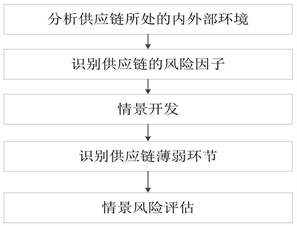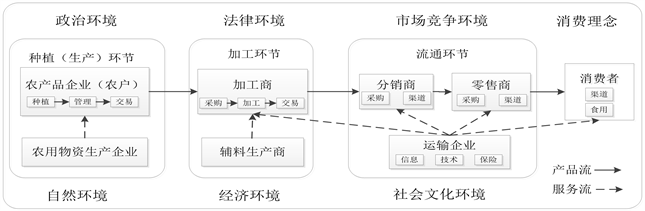# 基于情景分析的食品供应链质量安全风险研究Research on Quality and Safety Risk of Food Supply Chain Based on Scenario Analysis

• 全文下载: PDF(447KB)    PP.392-401   DOI: 10.12677/SD.2019.93048
• 下载量: 188  浏览量: 810

In recent years, food quality and safety issues have received much attention. Food quality and safety risks have a bad impact on social security and stability, sustainable development of the industry, and healthy living of residents, increasing the possibility of loss of interests of all parties and monitoring the quality and safety risks of food supply chains. The difficulty stems from the uncertainty of the supply environment, the complexity of the food supply chain, and the conduc-tivity of each link. The article introduces the Scenario Analysis method into the research of the quality and safety risks of the food supply chain. The supply chain environment is safely differen-tiated into 8 different scenarios, and the risk identification of three typical scenarios is obtained, and the scenario-based risk combination is obtained. The fuzzy comprehensive evaluation and the G1 method are used to evaluate the risk combination, and the risk loss under different scenarios is quantified. Risk response measures under different scenarios have certain guiding significance for grasping the key control points of food supply chain quality and safety risks.

1. 引言

2. 文献综述Figure 1. Scenario-based supply chain risk assessment

3. 基于情景分析的食品供应链质量安全风险管理

3.1. 食品供应链结构以及内外部环境Figure 2. Food supply chain structure

3.2. 食品供应链质量安全风险因子Table 1. The whole process safety risk factor of the food supply chain

3.3. 情景开发Table 2. Food Supply Chain Scenario Analysis Matrix

3.4. 食品供应链薄弱环节识别

4. 基于情景的食品供应链质量安全风险模糊综合评价

4.1. 确定评语集Table 3. Vulnerability assessment

4.2. 确定指标权重

G1法是东北大学郭亚军教授提出的一种方法，它通过对AHP进行改进，避开了AHP中的缺点，而且该方法无需一致性检验 。以情景1为例，运用G1法确定风险指标的权重：

1) 确定序关系：由5位专家对指标评分的均值大小得到情景1的风险因子指标序关系： ${U}_{34}\succ {U}_{32}\succ {U}_{21}\succ {U}_{52}\succ {U}_{31}\succ {U}_{33}\succ {U}_{43}\succ {U}_{42}\succ {U}_{51}\succ {U}_{22}$ ，记为： ${X}_{1}\succ {X}_{2}\succ {X}_{3}\succ {X}_{4}\succ {X}_{5}\succ {X}_{6}\succ {X}_{7}\succ {X}_{8}\succ {X}_{9}\succ {X}_{10}$

2) 确定相邻指标之间的相对重要程度：专家对相邻指标 ${X}_{k-1}$${X}_{k}$ 之间的重要程度之比可以使用： ${r}_{k}={\omega }_{k-1}/{\omega }_{k}，k=m,m-1,m-2,\cdots ,3,2$ 来表示，其中 ${r}_{k}$ 的取值可由相邻指标的评分均值之比得到。表4${r}_{k}$ 取值说明。

3) 计算指标的权重：明显有， ${r}_{k-1}\ge {r}_{k}$$k=m,m-1,m-2,\cdots ,3,2$ 则第k个指标的权重计算公式为：

${\omega }_{m}={\left[1+{\sum }_{k=2}^{m}{\prod }_{i=k}^{m}{r}_{i}\right]}^{-1}$(1)

${\omega }_{k-1}={r}_{k}{\omega }_{k},k=m,m-1,m-2,\cdots ,3,2$ (2)Table 4. A description of the relative importance between the indicators

$\begin{array}{c}{\omega }_{1}=\left({\omega }_{21},{\omega }_{22},{\omega }_{31},{\omega }_{32},{\omega }_{33},{\omega }_{34},{\omega }_{42},{\omega }_{43},{\omega }_{51},{\omega }_{52}\right)\\ =\left(0.1150,0.0752,0.1018,0.1195,0.1018,0.1239,0.0841,0.0885,0.0796,0.1106\right)\end{array}$

$\begin{array}{c}{\omega }_{2}=\left({\omega }_{11},{\omega }_{12},{\omega }_{13},{\omega }_{22},{\omega }_{31},{\omega }_{32},{\omega }_{34},{\omega }_{41},{\omega }_{42},{\omega }_{43},{\omega }_{51},{\omega }_{52}\right)\\ =\left(0.1007,0.0972,0.0868,0.0694,0.0868,0.0938,0.0972,0.0764,0.0660,0.0729,0.0833,0.0694\right)\end{array}$

$\begin{array}{c}{\omega }_{3}=\left({\omega }_{11},{\omega }_{12},{\omega }_{13},{\omega }_{21},{\omega }_{22},{\omega }_{23},{\omega }_{31},{\omega }_{32},{\omega }_{33},{\omega }_{34},{\omega }_{35},{\omega }_{41},{\omega }_{42},{\omega }_{43},{\omega }_{51},{\omega }_{52},{\omega }_{53}\right)\\ =\left(0.0808,0.0651,0.0677,0.0729,0.0495,0.0521,0.0625,0.0780,0.0625,\\ \text{\hspace{0.17em}}\text{\hspace{0.17em}}0.0755,0.0443,0.0443,0.0391,0.0573,0.0417,0.0599,0.0469\right)\end{array}$

4.3. 确定模糊综合判断矩阵

${R}_{i}=\left[\begin{array}{cccc}{r}_{11}& {r}_{12}& \cdots & {r}_{15}\\ {r}_{21}& {r}_{22}& \cdots & {r}_{25}\\ ⋮& ⋮& \ddots & ⋮\\ {r}_{m1}& {r}_{m2}& \cdots & {r}_{m5}\end{array}\right]$

4.4. 综合评判结果

5. 总结与建议

NOTES

*通讯作者。

  刘永胜. 食品供应链风险相关概念辨析[J]. 经济问题, 2014(8): 12-15.  宁钟, 王雅青. 基于情景分析的供应链风险识别——某全球性公司案例分析[J]. 工业工程与管理, 2007, 11(2): 88-94.  Heijden, K. (1996) The Art of Strategic Conversation. John Wiley &Sons Ltd., Hoboken.  陈娟, 张清楠. 食品供应链安全风险管理水平影响因素实证分析[J]. 商业经济研究, 2016(5): 27-29.  陈静彬. 基于熵值法和灰色关联分析的粮食安全预警研究——以湖南省为例[J]. 求索, 2009(8): 18-20.  朱淀, 洪小娟. 2006-2012年间中国食品安全风险评估与风险特征研究[J]. 中国农村观察, 2014(2): 49-59.  樊星, 邵举平, 孙延安. 基于模糊理论的跨国农产品供应链风险识别与评估[J]. 科技管理研究, 2016(6): 210-215.  郝世绵, 汪伟忠, 申慢慢. 食品全产业链三维风险评价[J]. 统计与决策, 2017(11): 38-41.  姚琪. 物联网环境下的食品供应链风险研究[J]. 食品工业, 2018, 39(5): 282-286.  邹俊. 食品安全供应链的透明度和诚信风险评价体系构建[J]. 商业经济研究, 2018(4): 28-30.  曾忠禄, 张冬梅. 不确定环境下解读未来的方法: 情景分析法[J]. 情报杂志, 2005(5): 14-16.  宁钟, 孙薇, 石香妍. 供应链风险的情景分析与管理[J]. 物流科技, 2006, 29(135): 56-60.  朱传波, 季建华,刘彩虹. 供应中断风险应急情景范式研究[J]. 上海管理科学, 2014, 36(4): 64-66.  莎娜, 季建华, 陈祥国. 供应链不确定性的情景分析探讨[J]. 情报杂志, 2011, 30(2): 194-198.  晚春东, 王娅, 索君莉. 供应链环境下食品质量安全风险问题研究[J]. 哈尔滨工业大学学报(社会科学版), 2014, 16(6): 136-140.  宁钟, 王雅倩. 基于亚太地区类SARS传染性疾病流行情景的供应链风险识别[J]. 科技导报, 2006, 24(8): 44-49.  林萍, 黄卫东, 张冲. 基于产品网络舆情话题的供应链风险特征研究[J]. 情报杂志, 2017, 36(12): 113-118.  丁斌, 孙政晓, 桂斌. 基于粗糙集与未确知模型的供应商风险评估方法研究[J]. 中国管理科学, 2008, 16(S1): 507-513.  宋宝娥, 马天山. 基于熵权和TOPSIS 的食品供应链可持续性评价研究[J]. 食品与生物技术学报, 2014, 33(7): 770-776.  陈敬贤, 施国洪, 马汉武. 基于改进灰关联分析法的供应链风险评价模型及应用研究[J]. 软科学, 2008, 22(10): 11-15.  王新利. 基于BP神经网络专家系统的供应链风险评价研究[J]. 中国流通经济, 2010, 24(6): 27-30.  郭亚军. 综合评价理论与方法[M]. 北京: 科学出版社, 2002.